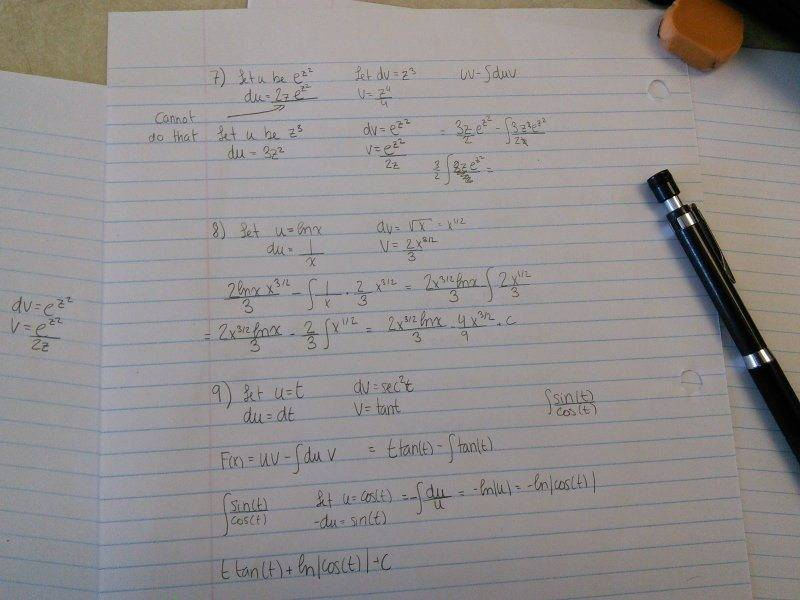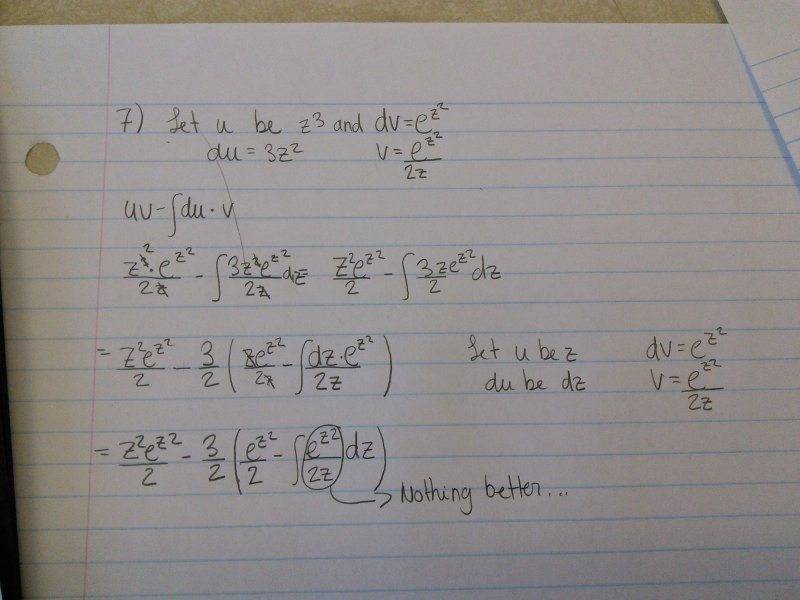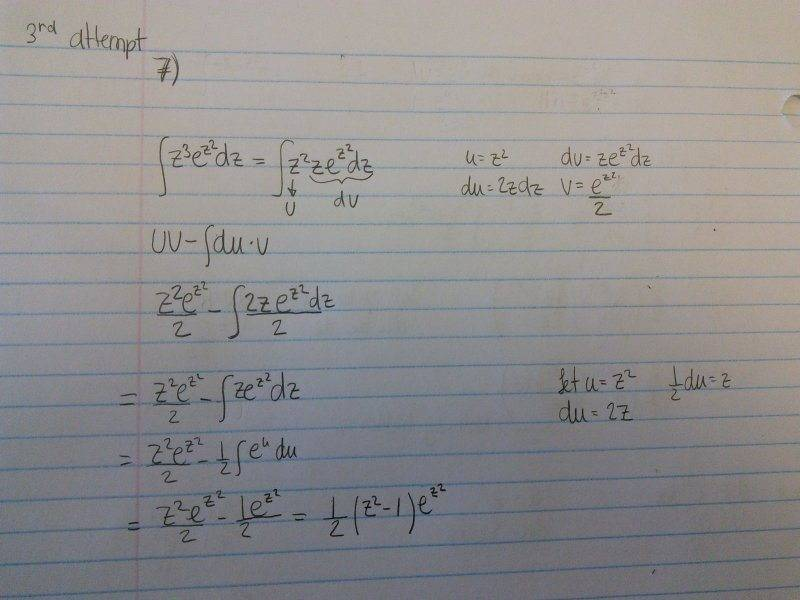# Need Help Doing Integation by Parts

Member warned about not showing an attempt

## Homework Statement

Find the integral of z^3 e^z^2

## Homework Equations

The integration by part formula

## The Attempt at a Solution

I have no idea what to do, I'm just turning in circles

SteamKing
Staff Emeritus
Homework Helper

## Homework Statement

Find the integral of z^3 e^z^2

## Homework Equations

The integration by part formula

## The Attempt at a Solution

I have no idea what to do, I'm just turning in circles
Well, show us your latest turn around the circle in working out this integral. You may have overlooked something simple.

Some integration by parts problems require using it a few times :D

Here's my attempt that doesn't work...And another oneIf dv = e^(z^2), v is not e^(z^2)/z^2. You cannot simply reverse the chain rule going backwards because if you take the derivative of that, you'll get something different from the quotient rule.

So when doing parts and one way doesn't work, what to do next?

Wel, normally you try going the other way around, but it still doesn't work as shown in the first picture...

SteamKing
Staff Emeritus
Homework Helper
If you are going to make dv = ez2 dz, then u = z3.

However, it is easier to integrate ez2 dz if you analyze it first using a u-substitution for z2.

Hint: you may not want to make u = z3 for this integral.

Oh ok! I think I get now! Thank you to everybody on this thread for your help! I'll try that!

Mark44
Mentor
And when you post again, @Airp, be sure to include at least some of what you have tried.

This is what I finally did! Thank you again!Mark44
Mentor
Also, it's better to include your work right here in the form rather than an image of it. Everything you wrote on paper can be done right here using LaTeX, which isn't really that difficult.

Here is one of the lines from the last image you posted.
$$\frac{z^2e^{z^2}}{2} - \int \frac{2ze^{z^2}dz}{2}$$

The LaTeX script before it is rendered looks like this:
\frac{z^2e^{z^2}}{2} - \int \frac{2ze^{z^2}dz}{2}
Note that I put an extra space between each pair of \$ symbols. That prevents the browser from rendering the script.

Fractions: \frac{}{}, with numerator in first pair of braces, and denominator in the second pair
Exponents: Use ^{} after the thing being raised to the power. If the exponent is a single character, the braces aren't needed.
Integrals (indefinite): \int
Integrals (definite): \int_a^b --Here a is the lower limit and b is the upper limit